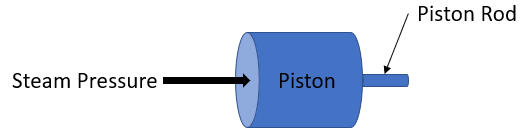## Steam Engine Piston

Consider a steam engine's piston has a 75 cm diameter, and a max steam gauge pressure of 2.0 MPa. If the piston's rod has a design stress of 70 MPa, what should be its minimum cross sectional area in m^2?Hint
$$Pressure =\frac{Force}{Area}$$$Hint 2 Since the piston force is the same force on the piston's rod, uniaxial loading is applied: $$\sigma =\frac{F}{A}$$$
where $$\sigma$$ is the stress on the cross section, $$F$$ is the loading, and $$A$$ is the cross sectional area.
Given the problem’s knowns, let's first find the force on the piston caused by the steam pressure.
$$\sum F=(pressure)(area)=(P)(\pi \cdot r^2)$$$$$F_{piston}=(2\cdot 10^6Pa)[\pi (.75/2m)^2]=883,125\:N$$$
Since the piston force is the same force on the piston's rod, uniaxial loading is applied:
$$\sigma =\frac{F}{A}\rightarrow F=\sigma A=F_{rod}$$$where $$\sigma$$ is the stress on the cross section, $$F$$ is the loading, and $$A$$ is the cross sectional area. Because $$F_{piston}=F_{rod}$$ , to solve for $$A_{rod}$$ : $$A_{rod}=\frac{F_{rod}}{\sigma}=\frac{883,125N}{70\cdot 10^6Pa}=0.013\:m^2$$$
$$0.013\:m^2$$\$